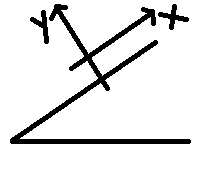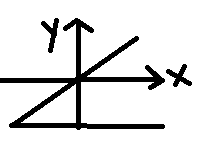# In newtons law of motion, while equating the normal force and weight (mg) in an inclined pane, inclined at an angle 30 degree to the horizontal, we use the equation mgcos30=N (normal reaction). My question is that why dont we equate mg with any component N (normal reaction)11 years ago

Your question makes sense...but it is done to simplify our calculations.

acceleration of the body is down the plane i.e. it is at an angle with the horizontal. if we change the coordinate axis in accordance with the direction of acceleration we need to split only mg into its components.but, if we take the general cartesian axis, we need to spilt N, frictional forca and acceleartion into their respective components.this can be a tedious task..but if you are comfortable with it..its no harm doing that.All the best!

11 years ago

normal force acts with some angle

11 years ago

Because we do not know normally the NORMAL acting on the block...We only know the mass so we use its component to balance...and Thats it :)